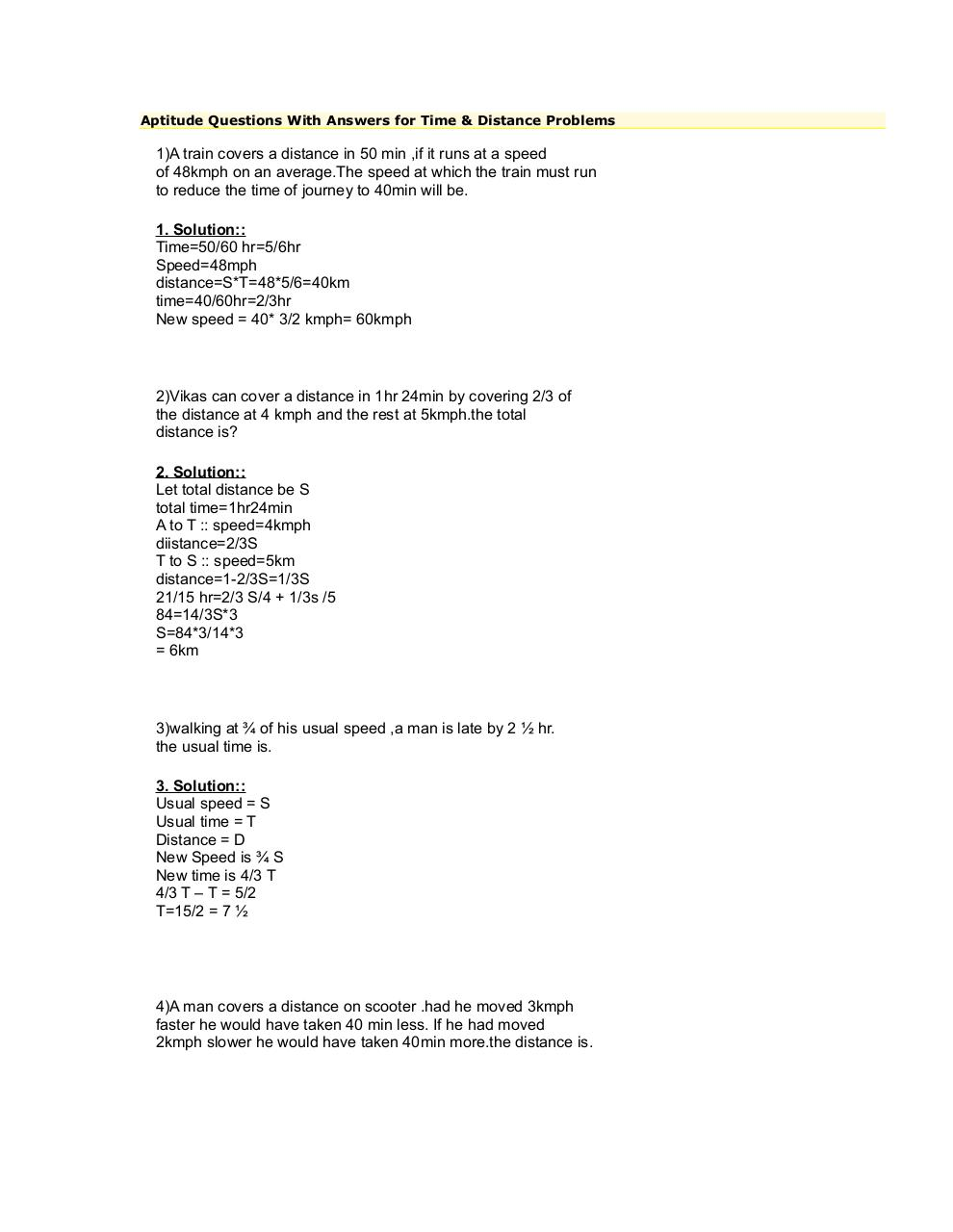# PDF Archive

Easily share your PDF documents with your contacts, on the Web and Social Networks.Page 12372

#### Text preview

Aptitude Questions With Answers for Time &amp; Distance Problems

1)A train covers a distance in 50 min ,if it runs at a speed
of 48kmph on an average.The speed at which the train must run
to reduce the time of journey to 40min will be.
1. Solution::
Time=50/60 hr=5/6hr
Speed=48mph
distance=S*T=48*5/6=40km
time=40/60hr=2/3hr
New speed = 40* 3/2 kmph= 60kmph

2)Vikas can cover a distance in 1hr 24min by covering 2/3 of
the distance at 4 kmph and the rest at 5kmph.the total
distance is?
2. Solution::
Let total distance be S
total time=1hr24min
A to T :: speed=4kmph
diistance=2/3S
T to S :: speed=5km
distance=1-2/3S=1/3S
21/15 hr=2/3 S/4 + 1/3s /5
84=14/3S*3
S=84*3/14*3
= 6km

3)walking at ¾ of his usual speed ,a man is late by 2 ½ hr.
the usual time is.
3. Solution::
Usual speed = S
Usual time = T
Distance = D
New Speed is ¾ S
New time is 4/3 T
4/3 T – T = 5/2
T=15/2 = 7 ½

4)A man covers a distance on scooter .had he moved 3kmph
faster he would have taken 40 min less. If he had moved
2kmph slower he would have taken 40min more.the distance is.